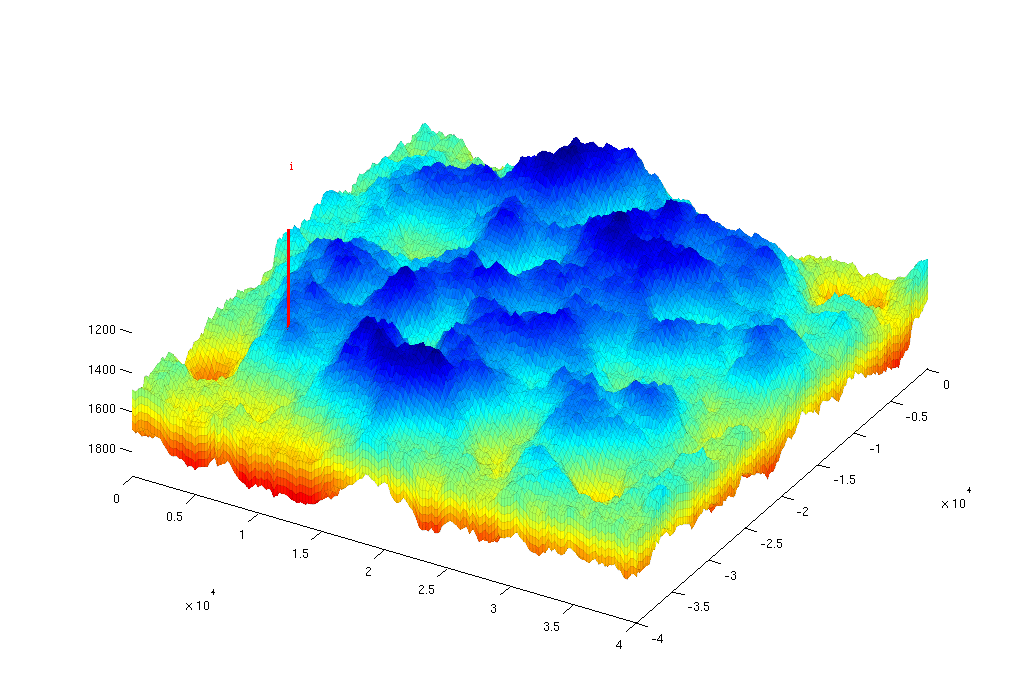#VE Simulation: Realistic Synthetic Aquifer

In this example we consider a synthetic sloping aquifier created in the IGEMS-CO2 project. The dataset in this example is available for download on this page.

We demonstrate the use of C-accelerated MATLAB, using the functions

• processgrid (replaces processGRDECL)
• mcomputegeometry (replaces computeGeometry)

see the release notes of MRST 2011a for details.

## Construct stratigraphic and petrophysical model

```addpath(fullfile(ROOTDIR,'mex','libgeometry'));

grdecl = readGRDECL(fullfile(ROOTDIR, 'data', 'igems', ...
'IGEMS.GRDECL'));

% Map from left-hand to right-hand coordinate system and process grid
lines = reshape(grdecl.COORD,6,[]);
lines([2 5],:) = -lines([2 5],:);
grdecl.COORD = lines(:); clear lines
G = processgrid(grdecl); toc

% Compute geometry, adding tags needed by topSurfaceGrid
G = mcomputeGeometry(G); toc
G.cells.faces = [G.cells.faces, repmat((1:6).', [G.cells.num, 1])];
rock = grdecl2Rock(grdecl);
rock.perm = convertFrom(rock.perm, milli*darcy);
clear grdecl

% Top-surface grid
disp(' -> Constructing top-surface grid');
[Gt, G] = topSurfaceGrid(G);
rock2D  = averageRock(rock, Gt);
```

## Set time and fluid parameters

```T          = 750*year();
stopInject = 150*year();
dT         = 1*year();
dTplot     = 2*dT;

% Make fluid structures, using data that are resonable at p = 300 bar
fluidVE = initVEFluid(Gt, 'mu' , [0.056641 0.30860] .* centi*poise, ...
'rho', [686.54 975.86] .* kilogram/meter^3, ...
'sr', 0.2, 'sw', 0.1, 'kwm', [0.2142 0.85]);
gravity on
```

## Set well and boundary conditions

We use one well placed down the flank of the model, perforated in the bottom layer. Injection rate is 2.8e4 m^3/day of supercritical CO2. Hydrostatic boundary conditions are specified on all outer boundaries.

```% Set well in 3D model
wellIx = [G.cartDims(1:2)/5, G.cartDims([3 3])];
rate   = 2.8e4*meter^3/day;
W      = verticalWell([], G, rock, wellIx(1), wellIx(2), ...
wellIx(3):wellIx(4), 'Type', 'rate', 'Val', rate, ...
'Radius', 0.1, 'comp_i', [1,0], 'name', 'I');

% Well in 2D model
wellIxVE = find(Gt.columns.cells == W(1).cells(1));
wellIxVE = find(wellIxVE - Gt.cells.columnPos >= 0, 1, 'last' );
WVE      = addWell([], Gt, rock2D, wellIxVE, ...

% BC in 2D model
i = any(Gt.faces.neighbors==0, 2);  % find all outer faces
I = i(Gt.cells.faces(:,1));         % vector of all faces of all cells, true if outer
j = false(6,1);                     % mask, cells can at most have 6 faces,
j(1:4)=true;                        %   extract east, west, north, south
J = j(Gt.cells.faces(:,2));         % vector of faces per cell, true if E,W,N,S
bcIxVE = Gt.cells.faces(I & J, 1);
bcVE = addBC([], bcIxVE, 'pressure', ...
Gt.faces.z(bcIxVE)*fluidVE.rho(2)*norm(gravity));
bcVE = rmfield(bcVE,'sat');
bcVE.h = zeros(size(bcVE.face));

% for 2D/vertical average, we need to change defintion of the wells
for i=1:numel(WVE)
WVE(i).compi=NaN;
WVE(i).h = Gt.cells.H(WVE(i).cells);
end
```

## Create figure and plot height and well

```scrsz  = get(0,'ScreenSize');
figVE = figure('Position',[scrsz(3)-1024 scrsz(4)-700 1024 700]);
set(0,'CurrentFigure',figVE);
plotCellData(G,G.cells.centroids(:,3),'EdgeColor','k','EdgeAlpha',0.05);
view([30 60]), axis tight;
plotWell(G, W, 'height', 1000, 'color', 'r'); drawnow
```## Prepare simulations

Compute inner products and instantiate solution structure

```SVE = computeMimeticIPVE(Gt, rock2D, 'Innerproduct','ip_simple'); toc
preComp = initTransportVE(Gt, rock2D);
sol = initResSol(Gt, 0);
sol.wellSol = initWellSol(W, 300*barsa());
sol.h = zeros(Gt.cells.num, 1);
sol.max_h = sol.h;
```

## Prepare plotting

We will make a composite plot that consists of several parts:

• a 3D plot of the plume
• a pie chart of trapped versus free volume
• a plane view of the plume from above
• two cross-sections in the x/y directions through the well

The code for the plotting is given here, and is also given in the full tutorial ` runIGEMS.m ` in the VE module.

## Main loop

Run the simulation using a sequential splitting with pressure and transport computed in separate steps. The transport solver is formulated with the height of the CO2 plume as the primary unknown and the relative height (or saturation) must therefore be reconstructed.

```t = 0;

while t% Advance solution: compute pressure and then transport
sol = solveIncompFlowVE(sol, Gt, SVE, rock, fluidVE, ...
'bc', bcVE, 'wells', WVE);
sol = explicitTransportVE(sol, Gt, dT, rock, fluidVE, ...
'bc', bcVE, 'wells', WVE, 'preComp', preComp, 'intVert', false);

% Reconstruct 'saturation' defined as s=h/H, where h is the height of
% the CO2 plume and H is the total height of the formation
sol.s = height2Sat(sol, Gt, fluidVE);
assert( max(sol.s(:,1))<1+eps && min(sol.s(:,1))>-eps );
t = t + dT;

% Check if we are to stop injecting
if t>= stopInject
WVE  = []; bcVE = [];
end

% Compute trapped and free volumes of CO2
freeVol = ...
sum(sol.h.*rock2D.poro.*Gt.cells.volumes)*(1-fluidVE.sw);
trappedVol = ...
sum((sol.max_h-sol.h).*rock2D.poro.*Gt.cells.volumes)*fluidVE.sr;
totVol = trappedVol + freeVol;

% Plotting (...) see the full tutorial runIGEMS.m in the VE module for details.

end
```

Published March 4, 2011